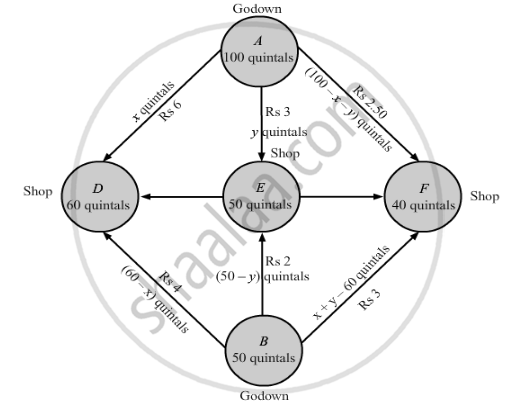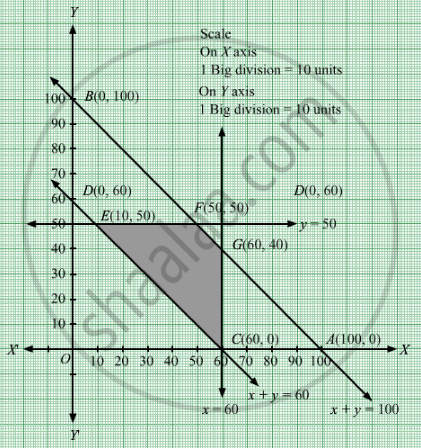# Tow Godowns, a and B, Have Grain Storage Capacity of 100 Quintals and 50 Quintals Respectively.They Supply to 3 Ration Shops, D, E and F, Whose Requirements Are 60, 50 and 40 Quintals Respectively. - Mathematics

Sum

Tow godowns, A and B, have grain storage capacity of 100 quintals and 50 quintals respectively. They supply to 3 ration shops, DE and F, whose requirements are 60, 50 and 40 quintals respectively. The cost of transportation per quintal from the godowns to the shops are given in the following table:

 Transportation cost per quintal(in Rs.) From-> A B To D 6.00 4.00 E 3.00 2.00 F 2.50 3.00

How should the supplies be transported in order that the transportation cost is minimum?

#### Solution

Let godown A supply x quintals and y quintals of grain to the shops D and E respectively.
Then, (100 − x − y) will be supplied to shop F.

The requirement at shop D is 60 quintals since, x quintals are transported from godown A.
Therefore, the remaining (60 − x) quintals will be transported from godown B.

Similarly, (50 − y) quintals and 40 − (100 − − y) i.e. (x + y − 60) quintals will be transported from godown B to shop E and F respectively.

The given problem can be represented diagrammatically as follows.Quantity of the grain cannot be negative.Therefore,

x ≥ 0 , y ≥ 0 and 100 - x - y ≥ 0

⇒ x ≥ 0 , y ≥ 0 , and x + y ≤ 100

60 - x ≥ 0 , 50 - y ≥ 0 , and x + y - 60 ≥ 0

⇒ x ≤ 60 , y ≤ 50 , and x + y ≥ 60

Total transportation cost Z is given by,

$Z = 6x + 3y + 2 . 5\left( 100 - x - y \right) + 4\left( 60 - x \right) + 2\left( 50 - y \right) + 3\left( x + y - 60 \right)$
$= 6x + 3y + 250 - 2 . 5x - 2 . 5y + 240 - 4x + 100 - 2y + 3x + 3y - 180$
$= 2 . 5x + 1 . 5y + 410$

The given problem can be formulated as:

Minimize Z = 2.5x + 1.5y + 410

subject to the constraints,

$x + y \leq 100$

$x \leq 60$

$y \leq 50$

$x + y \geq 60$

$x, y \geq 0$

First we will convert inequations into equations as follows:
x + y = 100, x = 60, y = 50, y =60, x = 0 and y = 0

Region represented by x + y ≤ 100:
The line x + y = 100 meets the coordinate axes at A1(100, 0) and B1(0, 100) respectively. By joining these points we obtain the line x + y = 100. Clearly (0,0) satisfies the x + y = 100. So, the region which contains the origin represents the solution set of the inequation x + y ≤ 100.

Region represented by x ≤ 60:
x = 60 is the line that passes (60, 0) and is parallel to the Y axis.The region to the left of the line = 60 will satisfy the inequation x ≤ 60.

Region represented by y ≤ 50:
y = 50 is the line that passes (0, 50) and is parallel to the X axis.The region below the line = 50 will satisfy the inequation y ≤ 50.

Region represented by x y ≥ 60:
The line x + y = 60 meets the coordinate axes at C1(60, 0) and $D_1 \left( 0, 60 \right)$ respectively. By joining these points we obtain the line x + y = 60. Clearly (0,0) does not satisfies the inequation x y ≥ 60. So,the region which does not contain the origin represents the solution set of the inequation x y ≥ 60.

Region represented by ≥ 0 and y ≥ 0:
Since, every point in the first quadrant satisfies these inequations. So, the first quadrant is the region represented by the inequations x ≥ 0, and y ≥ 0.

The feasible region determined by the system of constraints x + y ≤ 100, x ≤ 60, y ≤ 50, x y ≥ 60, x ≥ 0 and y ≥ 0 are as follows.The corner points are C1(60, 0), G1(60, 40), F1(50, 50), and E1(10, 50).

The values of Z at these corner points are as follows.

 Corner point Z = 2.5x + 1.5y + 410 C1(60, 0) 560 G1(60, 40) 620 F1(50, 50) 610 E1(10, 50) 510

The minimum value of Z is 510 at E1(10, 50).

Thus, the amount of grain transported from A to D, E, and F is 10 quintals, 50 quintals, and 40 quintals respectively and from B to DE, and F is 50 quintals, 0 quintals, and 0 quintals respectively.

The minimum cost is Rs 510.

Concept: Graphical Method of Solving Linear Programming Problems
Is there an error in this question or solution?

#### APPEARS IN

RD Sharma Class 12 Maths
Chapter 30 Linear programming
Exercise 30.5 | Q 1 | Page 65